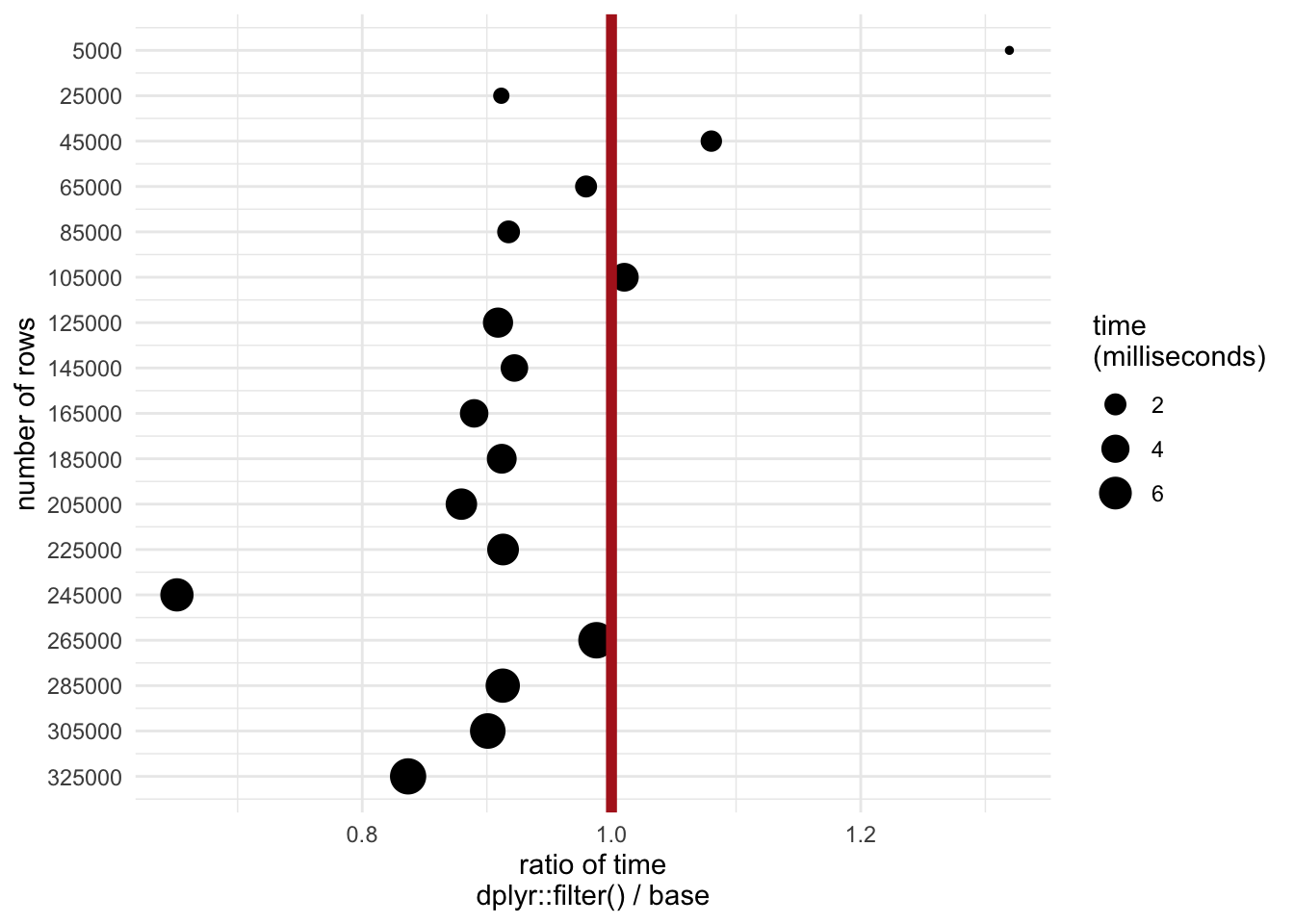# dplyr filter() Benchmark

Last week I finished preparing new teaching material for a workshop on R optimization techniques. Some of the examples were motivated by benchmarking tests I ran on data manipulation methods with `base` vs `dplyr` vs `data.table`.

Not surprisingly, `data.table` was generally most efficient in terms of speed when running the `microbenchmark` tests.

One oddball result did creep up …

When comparing `dplyr::filter()` versus a base method (using bracket indices and `which()`), I noticed that the former was quite a bit slower for a data frame with 5000 rows. But that result reversed as the size of the data frame increased … in other words, `filter()` was actually much faster for a larger dataset.

First we can load the packages we’ll be using:

``````library(microbenchmark)
library(dplyr)
library(ggplot2)
library(nycflights13)``````

Next create a sample of n = 5000 rows of the `nycflights13` dataset:

``````flights_sample <-
sample_n(flights, 5000)``````

Then run the benchmark on the sample of n = 5000 rows:

``````# run benchmark on n = 5000
microbenchmark(
bracket = flights_sample[which(flights_sample\$dep_delay > 0),],
filter = filter(flights_sample, dep_delay > 0),
times = 10
)``````
``````## Unit: microseconds
##     expr     min      lq     mean   median      uq     max neval
##  bracket 223.183 246.329 310.3268 290.7345 315.310 632.726    10
##   filter 265.342 352.911 387.6371 371.1365 382.088 673.066    10``````

Now run the benchmark on the full n = 336776 rows:

``````microbenchmark(
bracket = flights[which(flights\$dep_delay > 0),],
filter = filter(flights, dep_delay > 0),
times = 10
)``````
``````## Unit: milliseconds
##     expr      min       lq     mean   median       uq      max neval
##  bracket 8.844108 9.041099 12.35393 12.58248 13.52227 20.33769    10
##   filter 8.001824 8.159017 12.65863 12.27404 13.27080 21.73494    10``````

My guess is that there’s some overhead to using `filter()` … but this clearly pays off eventually. So how big (i.e. how many rows) does a data frame need to be for `filter()` to be faster?

``````# create sequence of number of rows to sample by 20000
sample_seq <- seq(5000, nrow(flights), by = 20000)

# set up empty data frame that will store results
mbm <- data_frame()

# loop through the samples and calculate microbencharmk in ms
for(n in sample_seq) {

flights_sample <- sample_n(flights, n)

tmpmbm <-
microbenchmark(
bracket = flights_sample[which(flights_sample\$dep_delay > 0),],
filter = filter(flights_sample, dep_delay > 0),
times = 10,
unit = "ms"
)

mbm <- rbind(mbm, summary(tmpmbm))

}

# add the sample n as a column for plotting
mbm\$n <- rep(sample_seq, each = 2)``````

The plot below shows the threshold (number of rows on y axis) at which `filter()` is faster (ratio < 1 on x axis). It also demonstrates that the absolute amount of time starts leveling off as the datasets get bigger.

``````mbm %>%
select(method = expr,
nrows = n,
time = median) %>%
group_by(nrows) %>%
mutate(ratio = time / lag(time)) %>%
filter(!is.na(ratio)) %>%
ggplot(aes(nrows, ratio, size = time)) +
geom_point() +
geom_hline(yintercept = 1, col = "firebrick", lwd = 2) +
scale_x_reverse(breaks = sample_seq) +
xlab("number of rows") +
ylab("ratio of time\ndplyr::filter() / base") +
coord_flip() +
theme_minimal() +
guides(size = guide_legend(title = "time\n(milliseconds)"))``````